# Difference between revisions of "RealisticAcceleration"

Here you'll find out how exactly the train acceleration is calculated if you enable realistic acceleration (see New Curve and Mountain Handling).

This realistic model takes into account physical properties of the train, such as engine weight, total train weight, power and tractive effort. It is of course not 100% realistic, because it doesn't support details such as a realistic torque curve, powered wheel arrangements and bogie types (although vehicles defined by new graphics sets can set this correctly), and other things that TTD knows nothing about. It had to work within the description of engines offered by TTD. As such, it assumes that the engines always operate at optimum torque, and ignores the differences between steam, diesel and electric propulsion (for example that usually not all wheels of a steam engine are powered, unlike most electric engines. Again, this is not necessarily true for new vehicle sets).

The result is thus not perfectly realistic, but it is reasonably close. It's certainly close enough that the train acceleration "feels" more realistic. For example, effects such as friction and air drag (enhanced in tunnels) are taken into account. Therefore very heavy trains may not reach their stated top speed at all, or be able to make it up a steep hill. The acceleration also decreases the faster a train is, so that it will accelerate very quickly in the beginning, but then take a long time to reach its top speed, just like a real train.

The basis of the calculation is the maximum force that can be generated by the engine. This is using an idealistic model of constant power, or equivalently, constant torque, which is close enough to reality though, for most real engines: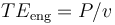$TE_\text{eng} = P / v$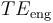$TE_\text{eng}$ is the maximum force generated by the engine in Kilonewtons, P is the power in Kilowatts and v is the speed in m/s. Of course the patch has to do a conversion from TTD's units.

After this, the patch calculates the maximum tractive effort that the engine can exert on the rails. This depends on its adhesive weight (i.e. the weight resting on powered axles), and the coefficient of friction on steel:$TE_\text{max} = W_\text{adh} \times \mu$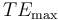$TE_\text{max}$ is the maximum tractive effort in kN,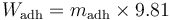$W_\text{adh} = m_\text{adh} \times 9.81$ is the total adhesive weight in kN, and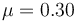$\mu = 0.30$ is the coefficient of friction for steel on steel as used by TTDPatch.

The actual tractive effort is then the lower of the two values: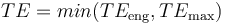$TE = min (TE_\text{eng}, TE_\text{max})$

This is then added up for all powered vehicles in the consist, which gives the total tractive effort shown in the vehicle speed window if you have enabled that, see below.

From the total tractive effort, the patch subtracts the loss forces of static/kinetic friction, rolling friction and air resistance, and any tangential forces from an inclined track to arrive at the net force: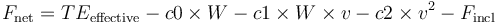$F_\text{net} = TE_\text{effective} - c0 \times W - c1 \times W \times v - c2 \times v^2 - F_\text{incl}$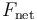$F_\text{net}$ is the net force in kN, W is the total weight of the train in kN, c0 is the coefficient of static/kinetic friction, c1 is the coefficient of rolling friction, c2 is the coefficient of air resistance and <math<F_\text{incl}[/itex] includes the tangential forces of inclined track.

For c0 and c1 the patch uses the same values for all trains. These values were chosen to give a realistic feeling to the acceleration, and not strictly derived. The reason for that is that TTD doesn't have a consistent time scale, so that it is impossible to calculate the values of such constants. c2 depends on the top speed of the engine, to account for the fact that faster engines are usually streamlined. Roughly speaking, an engine that has twice the top speed than another engine is considered to have half the air resistance.

The incline forces are calculated as a sum over all train vehicles: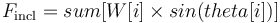$F_\text{incl} = sum [ W[i] \times sin(theta[i]) ]$

W[i] is the weight of the i'th vehicle and theta[i] is the slope of the incline for that vehicle.

The slope is taken to be either -5%, 0% or +5%, depending on whether the vehicle is moving downhill, flat or uphill.

Finally, once the net force is known, the patch can calculate the train acceleration: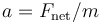$a = F_\text{net} / m$

a is the acceleration in m/s2 and m is the total mass of the train in kg.

And that's the final acceleration value used to calculate the increase in train speed from one engine tick to the next.

If you are interested, you can see the resulting values for tractive effort and acceleration by using custom patch texts, see Detailed Information. You need to modify the "headingfor" entries to have them show tractive effort and acceleration. For example, use the following format:

headingfor="\95\80, \84, TE=\7c kN, a=\7c\00"

This shows the tractive effort (TE) in Kilonewtons, and the acceleration (a) in cm/s². The unit of acceleration is cm/s² because it is usually very small, and TTD cannot easily show decimals. It is only used if "showspeed" is on. Note that this can sometimes make the status bar text too wide to fit in the window, if that annoys you, you will have to remove these displays again.